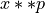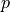# sfepy.mesh.mesh_generators module¶

sfepy.mesh.mesh_generators.gen_block_mesh(dims, shape, centre, mat_id=0, name='block', coors=None, verbose=True)[source]

Generate a 2D or 3D block mesh. The dimension is determined by the lenght of the shape argument.

Parameters
dimsarray of 2 or 3 floats

Dimensions of the block.

shapearray of 2 or 3 ints

Shape (counts of nodes in x, y, z) of the block mesh.

centrearray of 2 or 3 floats

Centre of the block.

mat_idint, optional

The material id of all elements.

namestring

Mesh name.

verbosebool

If True, show progress of the mesh generation.

Returns
meshMesh instance
sfepy.mesh.mesh_generators.gen_cylinder_mesh(dims, shape, centre, axis='x', force_hollow=False, is_open=False, open_angle=0.0, non_uniform=False, name='cylinder', verbose=True)[source]

Generate a cylindrical mesh along an axis. Its cross-section can be ellipsoidal.

Parameters
dimsarray of 5 floats

Dimensions of the cylinder: inner surface semi-axes a1, b1, outer surface semi-axes a2, b2, length.

shapearray of 3 ints

Shape (counts of nodes in radial, circumferential and longitudinal directions) of the cylinder mesh.

centrearray of 3 floats

Centre of the cylinder.

axis: one of ‘x’, ‘y’, ‘z’

The axis of the cylinder.

force_hollowboolean

Force hollow mesh even if inner radii a1 = b1 = 0.

is_openboolean

Generate an open cylinder segment.

open_anglefloat

non_uniformboolean

If True, space the mesh nodes in radial direction so that the element volumes are (approximately) the same, making thus the elements towards the outer surface thinner.

namestring

Mesh name.

verbosebool

If True, show progress of the mesh generation.

Returns
meshMesh instance
sfepy.mesh.mesh_generators.gen_extended_block_mesh(b_dims, b_shape, e_dims, e_shape, centre, grading_fun=None, name=None)[source]

Generate a 3D mesh with a central block and (coarse) extending side meshes.

The resulting mesh is again a block. Each of the components has a different material id.

Parameters
b_dimsarray of 3 floats

The dimensions of the central block.

b_shapearray of 3 ints

The shape (counts of nodes in x, y, z) of the central block mesh.

e_dimsarray of 3 floats

The dimensions of the complete block (central block + extensions).

e_shapeint

The count of nodes of extending blocks in the direction from the central block.

centrearray of 3 floats

The centre of the mesh.

A function ofthat can be used to shift nodes in the extension axis directions to allow smooth grading of element sizes from the centre. The default function iswithdetermined so that the element sizes next to the central block have the size of the shortest edge of the central block.

namestring, optional

The mesh name.

Returns
meshMesh instance
sfepy.mesh.mesh_generators.gen_mesh_from_geom(geo, a=None, verbose=False, refine=False)[source]

Runs mesh generator - tetgen for 3D or triangle for 2D meshes.

Parameters
geogeometry

geometry description

aint, optional

a maximum area/volume constraint

verbosebool, optional

detailed information

refinebool, optional

refines mesh

Returns
meshMesh instance

triangular or tetrahedral mesh

sfepy.mesh.mesh_generators.gen_mesh_from_string(mesh_name, mesh_dir)[source]
sfepy.mesh.mesh_generators.gen_mesh_from_voxels(voxels, dims, etype='q')[source]

Generate FE mesh from voxels (volumetric data).

Parameters
voxelsarray

Voxel matrix, 1=material.

dimsarray

Size of one voxel.

etypeinteger, optional

‘q’ - quadrilateral or hexahedral elements ‘t’ - triangular or tetrahedral elements

Returns
——-
meshMesh instance

Finite element mesh.

sfepy.mesh.mesh_generators.gen_misc_mesh(mesh_dir, force_create, kind, args, suffix='.mesh', verbose=False)[source]

Create sphere or cube mesh according to kind in the given directory if it does not exist and return path to it.

sfepy.mesh.mesh_generators.gen_tiled_mesh(mesh, grid=None, scale=1.0, eps=1e-06, ret_ndmap=False)[source]

Generate a new mesh by repeating a given periodic element along each axis.

Parameters
meshMesh instance

The input periodic FE mesh.

gridarray

Number of repetition along each axis.

scalefloat, optional

Scaling factor.

epsfloat, optional

Tolerance for boundary detection.

ret_ndmapbool, optional

If True, return global node map.

Returns
mesh_outMesh instance

FE mesh.

ndmaparray

Maps: actual node id –> node id in the reference cell.

sfepy.mesh.mesh_generators.get_tensor_product_conn(shape)[source]

Generate vertex connectivity for cells of a tensor-product mesh of the given shape.

Parameters
shapearray of 2 or 3 ints

Shape (counts of nodes in x, y, z) of the mesh.

Returns
connarray

The vertex connectivity array.

descstr

The cell kind.

sfepy.mesh.mesh_generators.main()[source]
sfepy.mesh.mesh_generators.tiled_mesh1d(conn, coors, ngrps, idim, n_rep, bb, eps=1e-06, ndmap=False)[source]